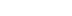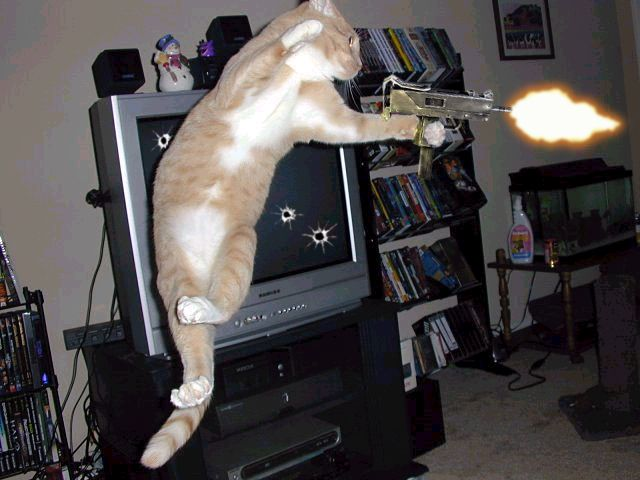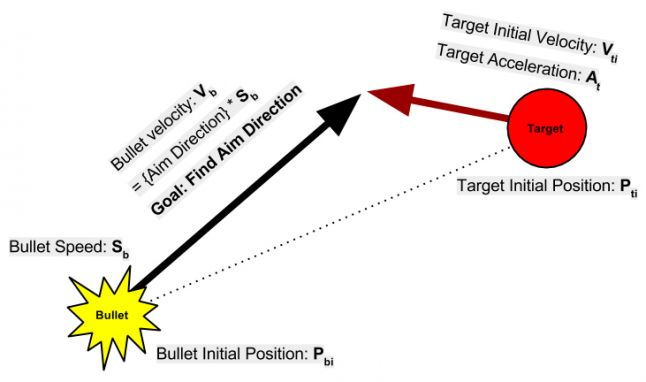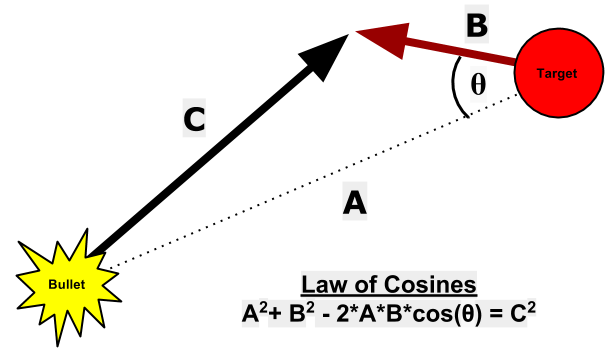/

Predictive Aim Mathematics for AI Targeting

A while back, I decided to look up algorithms for predictive firing on the internet, but I couldn't find any that incorporated acceleration in three dimensions, so I decided to give that the old college try.

UPDATE:

If you just want a function to paste/modify into your codebase, then go here for the Unity C# version:

Otherwise, feel free to jump to "Attempt #3" if you just want a quick answer to your search that puts you on the path to writing an informed utility function in your math library that you can call your very own.

I just found out that some folks are pointing others to this page as a reference. That is quite awesome! I am honored to have written something useful to the community at large. Feel free to add comments below or send me thoughts on ways to improve this article. Math corrections are also welcome. My favorite comments will be the ones where you tell me what game this helped you make :)

For the record, this is using math taught in high school/early college algebra, geometry, and physics. Teachers may sometimes find themselves faced with skeptical students wondering when they will ever use any of this stuff in real life:

• Kinematic Equations
• System of Equations + Degrees of Freedom
• Newton's Method
• Vector Properties
• Dot Product
• The Law of Cosines
• Exponent Properties

As a product of my education, I have a particular soft spot for math and science teachers. This page is my love letter to all of them.

A while back, I decided to look up algorithms for predictive firing on the internet, but I couldn't find any that incorporated acceleration in three dimensions, so I made an attempt at deriving the algorithm, myself.

This is my story. I hope you like it.Image Credit: The Internets (possibly from Something Awful's Photoshop Phriday)

The Problem:

Aim a projectile launcher to hit a moving target in n-dimensional space (2d or 3d) with a straight projectile of constant finite speed.

To keep this derivation simple, we can assume the projectile travels in a straight line and is immune to gravity. Once we have the straight line solution, we can modify that solution to support lobbed projectiles.

The Givens:

• Bullet Initial Position (i.e. muzzle position): Pbi
• Bullet Speed (scalar constant, finite): Sb
• Target Initial Position: Pti
• Target Initial Velocity: Vti
• Target Acceleration (constant): At

The Goal:

Find a unitized vector representing the bullet's firing direction. Since we know the bullet's speed as Sb, we can multiply that speed with the unit vector to get the vector that represents the bullet's velocity and direction.

For our purposes, we will combine Sb and this unknown direction into a single variable:

• Bullet velocity (constant vector): Vb = desiredAimDirection * Sb

The goal is to find the n-dimensional normalized vector, desiredAimDirection

Derivation Strategy:

Feel the Math...
Imagine two things happening at the same time:

• A sphere growing outward representing all possible places that a projectile can be at time t. Radius is 0 when t=0
• A line growing longer representing target position in relation to a starting target position from time t. Length is 0 when t=0

...Feel it!Become the NPC! When the enemy is before you, you see the matrix in your virtual simulation: the sphere growing outward from the muzzle of your weapon as your target attempts to flee in a straight line. In your virtual simulation, you see only the sphere centered at your muzzle and a line tracing from your target's head... and it is beautiful!

The rest of this page is an illustration of your NPC's sphere of potential influence intercepting the straight line defined by the target's current position and movement.

Philosophically and spiritually, we are seeking that position in time and space at which the arrowhead of that growing line from the target intersects the edge of that growing sphere from the muzzle.

I am intentionally not including a picture of an arrowed line and sphere here to encourage you to develop/confirm your own neural pathways to instinctively and flexibly form this time-based moving model in your head as a prerequisite for being able to understand how this solution works for any predictive aim scenario... "Open your mind, Quaid! Open your mind!!!"

Yay Physics!

We are all born with an inherent understanding of physics, which is why we know that the line representing target motion will grow in length over time.

The Kinematic Equations allow us to convert positions, time, and speed into growing lines and spheres. This is the kinematic equation relevant to our purpose:
PositionFinal = PositionInitial + VelocityInitial*time + 0.5*Acceleration*time2

If the perfectly aimed bullet meets the moving target, then we can say that finalPositions will coincide between the kinematic equations for the moving bullet and the moving target.

Note that I am using the word "bullet" instead of projectile in order to play nice with acronyms where P unambiguously means "Position" instead of "Projectile", and B means "Bullet". Apologies in advance for the ambiguity between time t and "Target".

• final position of bullet with zero acceleration = final position of target with nonzero acceleration
• Pbi + Vb*t = Pti + Vti*t + 0.5*At*t2

The thought journey goes as follows...

1. Finding 't' leads to knowing the final position of both, the projectile and the target
2. the final position is where the muzzle would need to aim.
3. The above equation can not be solved by itself because Vb is also unknown.

Strategy #1 - System of Equations with Acceleration:

There are two unknowns in the above equation: t and Vb.

Vb is a vector of n-dimensional velocity that is the result of the normalized vector of bullet heading times scalar magnitude of bullet speed, Sb.

Since there are two unknowns and only one equation, then one method of solving this is through a system of equations. With two independent equations and two unknowns, we can isolate one of the unknowns (like t) and plug that into the other equation to identify the other unknown (Vb). Knowing the value of Vb and Sb will allow us to determine the normalized vector that defines the desired heading of the muzzle when it shoots.

For the second equation, I specifically wanted to take direction out of the picture when it came to the bullet because I know its speed but not its direction. Without direction, the bullet can be thought of as a growing sphere. Hence, the other equation I used for this method feels like a parametric representation of the bullet as a spherical AoE (Area of Effect) field growing outward per t whose radius would be equivalent to the distance from muzzle to target at the proper value of t. So when t is 0, the radius of that sphere is 0, and when t is righteous, the distance from muzzle to target will intersect in one of two places... since lines are able to intersect spheres in two places.

Combining the two concepts, I had an equation that looked like this:

1. Distance from muzzle to target = distance traveled by the bullet in t seconds
2. Length( finalTargetPosition - muzzlePosition ) = distance traveled by the bullet in t seconds
3. Length(Pti + Vti*t + 0.5*At*t2 - Pbi) = Sb*t

Good news: Only one unknown in the above equation. If we isolate the t, then we can plug that t into this known equation:

• Pbi + Vb*t = Pti + Vti*t + 0.5*At*t2

...in order to isolate Vb, which will lead us to the goal.

Bad news: I did the math (which I'll go into detail with in Attempt#3), but gave up and then decided not to post my formulas in this article because it was uuuuuuugly. The generic solution to the combined equation involving Vector magnitude is technically doable, but an isolated t in terms of all those variables would end up being hairy to the 4th degree because of acceleration, which gives you t4 somewhere in that mix after doing Law of Cosines followed by Quadratic coefficient determination.

I decided to bail on that direction and, instead, went with an iterative Newtonian-like approximation.

Strategy #2 - Iterative Approximation with Acceleration:

If I find the value of t that coincides with perfect impact, then I can find where that impact would occur by plugging into the target's kinematic equation. That future position would be where the muzzle should point at when it fires the projectile.

Feel the Math

Think of this as bouncing back and fourth between time, t, and Target Position, Pt which are defined by equations that feed into each other. Bouncing back and fourth gets you closer and closer to convergence as each new Pt gets you a more accurate new t, which gets you a more accurate new Pt after that and so fourth until you feel that your iterated Pt accurately reflects the actual Pt at the future time of impact, t.

Baseline iteration:

A fair first approximation is to assume that the target is not moving. In this case, the value of t is the time it takes for the bullet to travel from muzzle to target's current position.

• t0 = Length(Pti - Pbi) / Sb

First iteration:

So now you have t0, which is a decent first guess at the time of impact. Plug that t0 into the target's kinematic equation to find its position when t0 seconds have passed:

• Pt1 = Pti + Vti*t0 + 0.5*At*t02

Now you have a new position for the target at the supposed moment of impact, time to calculate a new t with that new position.

• t1 = Length(Pt1 - Pbi) / Sb

Recurring Iteration:

Now you have a new time, t1. t1 is better than t0 because it took a more accurate position guess into account. If you want, you can go on as many times as you are willing to iterate to get an answer that is "good enough". Be sure to use the original target position Pti, as the kinematic equation is based on the original known position at time t=0.

• Pt2 = Pti + Vti*t1 + 0.5*At*t12
• t2 = Length(Pt2 - Pbi) / Sb

So the general pattern is...

• Pt[n] = Pti + Vti*(t[n-1]) + 0.5*At*t[n-1]2
• t[n] = Length(Pt[n] - Pbi) / Sb

And the answer you are looking for is...
desiredAimDirection = Normalize( Pt[n] - Pbi);
... to hit that target in t[n] seconds.

Assuming that the speed of the bullet is faster than the speed of the target (imagine a growing Area of Effect), the deltas between successive t and P values will diminish between each iteration. Those t values are mathematically bound to converge asymptotically upon the correct value of t depicting the time that projectile and target need to travel before reaching coinciding positions.

The nice thing about this iteration method is that it can be easier on CPU cycles if you arrange the code to amortize iteration steps across separate cycles as part of your AI CPU-load budgeting methods. One side effect of iteration is that using the value of t from your current step in the next iteration across ticks can make it look like your AI's aim is gradually becoming more accurate for targets that move predictably... as if they were refining their aim while looking at you :)

In the case of a first person shooter, you probably want AIs to aim their missed shots in front of the player target's camera for dramatic effect. This particular algorithm has enemy aim statistically likely to chase the moving target from behind.

Supporting Lobbed Projectiles for Strategy #2:

Lobbed projectiles are simply projectiles that have downward acceleration on them, assuming gravity goes down in your video game simulation :)

Spiritually, Equation 2 represents this:

1. finalProjectilePosition = finalTargetPosition
2. Gravity = Ab
3. Pbi + Vb*t[n] + 0.5*Ab*t[n]2 = Pt[n]
4. Vb*t[n] = Pt[n] - Pbi - 0.5*Ab*t[n]2
5. Vb = [ Pt[n] - Pbi - 0.5*Ab*t[n]2 ] / t[n]
6. Vb = [ Pt[n] - Pbi ] / t[n] - 0.5*Ab*t[n]

And the answer you are looking for is...
Vb
... to hit that target at Pt[n] in t[n] seconds.

Note that Vb is explicitly NOT Normalized in this solution. If something feels a little off about this, you are correct; we are basically saying that the magnitude of the bullet's initial velocity is affected by the angle of the lob. I will go into more detail on the relationship between gravity and initial bullet velocity after Strategy #3.

Strategy #3 - Assuming Zero Acceleration:

The downside of Strategy #2 is that it requires multiple cycles and even then, it is not exact. If you want to do this as a single pass utility function that is mathematically accurate on the first attempt, then we're going to have to revisit the original strategy described in #1.

Acceleration is what makes the Strategy #1 difficult to solve because it throws that t2 into the mix which eventually becomes a t4 when you convert that vector into a scalar magnitude.

The problem of predictive aim is much more solvable if we just drop this whole Acceleration bit.

For completeness sake, here is the derivation of predictive aim direction for a target moving with zero acceleration:

• Target Starting Velocity vector: Vti can now be just Vt since it is constant
• Equation 1: Length(finalTargetPosition - muzzlePosition) = distanceTraveledByProjectile
• Equation 1: Length(Pti + Vt*t - Pbi) = Sb*t
• Equation 2: finalProjectilePosition = finalTargetPosition
• Equation 2: Pbi + Vb*t = Pti + Vt*t

Two equations. Two unknowns, t and Vb

First find t, then you can use that to solve for Vb

Equation 1 can be broken down to isolate t through these simple steps:

1. Length(Pti - Pbi + Vt*t) = Sb*t
2. Length( (Pti - Pbi) + Vt*t) = Sb*t
3. theta is the angle between (Pti - Pbi) and Vt*t
4. If the target was moving at a perfect 90 degree angle from the muzzle (theta == 90 degrees), then the pythagorean theorem would have been enough, but the target is more likely to run at a skewed angle ranging from perfectly towards to perfectly away from the muzzle. And so we use the Law of Cosines, instead
5. Law of Cosines: A2+ B2 - 2*A*B*cos(theta) = C26. Law of Cosines:
• A = Length(Pti - Pbi)
• B = Length(Vt*t)
• C = Sb*t
7. Dot Product:
• cos(theta) = DotProduct( Normalize(Pbi - Pti), Normalize(Vt*t) )
• cos(theta) = DotProduct( Normalize(Pbi - Pti), Normalize(Vt) )
8. Length(Pti - Pbi)2 + Length(Vt*t)2 - 2*Length(Pti - Pbi)*Length(Vt*t)*cos(theta) = (Sb*t)2
9. Let’s refer to Length(Pti - Pbi) as D to represent initial distance from muzzle to target
10. D2 + Length(Vt*t)2 - 2*D*Length(Vt*t)*cos(theta) = (Sb*t)2
11. D2 + (Length(Vt)*t)2 - 2*D*Length(Vt)*t*cos(theta) = (Sb*t)2
12. Let’s refer to Length(Vt) as St to represent Target Speed
13. D2 + (St*t)2 - 2*D*St*t*cos(theta) = (Sb*t)2
14. D2 + St2*t2 - 2*D*St*t*cos(theta) = Sb2*t2
15. 0 = Sb2*t2 - D2 - St2*t2 + 2*D*St*t*cos(theta)
16. 0 = Sb2*t2 - St2*t2 + 2*D*St*t*cos(theta) - D2
17. 0 = (Sb2 - St2)*t2 + 2*D*St*cos(theta)*t - D2
18. Quadratic formula: For 0 = a*t2 + b*t + c
• a = (Sb2 - St2)
• b = 2*D*St*cos(theta)
• c = -D2
• t = [ -b ± Sqrt( b- 4*a*c ) ] / (2*a)
19. The dot product result from above is the value to use for cos(theta)
20. You can probably stop here to solve for t since you likely have those coefficients a, b, and c stored as local variables
21. t = [ -2*D*St*cos(theta) ± Sqrt[ (2*D*St*cos(theta))2 + 4*(Sb2 - St2)*D2 ] ] / (2*(Sb2 - St2))

You now have two possible values of t.
This can happen when you are intersecting a growing line with a growing sphere.

"But which one of you is the real t?"
"You're going to have to calculate us both... it's the only way!"

If t is negative, you can eliminate that value immediately because time-reversing computers will not be available to humans until the year 2942. By then, we will all be dead.

If both values of t are positive, then you may want to pick the smallest one if that is the design you are "shooting" for (get it? shooting? LOL). You basically have a situation where both possibilities will reach their mark (i.e. target is running away from your NPC at a skewed angle from a somewhat slow projectile), but you probably want the NPC to bias towards hitting sooner rather than later... perhaps. The choice is yours.

We want to isolate Vb in Equation 2: Pbi + Vb*t = Pti + Vt*t

1. Vb*t = Pti + Vt*t - Pbi
2. Vb = (Pti + Vt*t - Pbi) / t
3. Vb = Vt + [(Pti - Pbi) / t]

You can check your math by making sure that...
Length(Vb) == Sb

If your numbers are slightly off, then this may be attributed to floating point precision loss. The best advice I can give you on that is to...

1. Use double over float whenever possible
2. Favor multiplication over division to minimize loss of significant digits
3. Avoid using sqrt as much as possible to minimize loss of significant digits

Vb being an n-dimensional vector should get you the direction you need:
desiredAimDirection = Normalize( Vb );

Situations in which there will be no valid solution:

If the speed of the projectile is slower than or equal to the speed of the target, then there will be cases where hitting the target is just not possible because target can literally outrun the projectile at most angles. You will want to detect these cases in order to avoid NaN issues in the code.

You can detect these non-solution cases with the following tests:

1. If the speed of projectile and target are equal, valid solution is definitely not possible if theta is greater than or equal to 90 degrees, cos(theta) <= 0
2. If you get square root of a negative number, then that imaginary number also indicates no solution. Check your discriminant (b- 4*a*c ) for negative values
3. If both calculated values of t are less than 0, then that means valid solutions only exist if the projectile can travel through time, which can't possibly happen until the year 2942, when machines finally solve the "human problem".

Supporting Lobbed Projectiles for Strategy #3:

"finalProjectilePosition = finalTargetPosition"

If your projectiles are affected by gravity, then all you have to do is modify Equation 2 to account for constant projectile acceleration in 2D/3D space:
Pbi + Vb*t + 0.5*Ab*t2 = Pti + Vt*t

At this point, we have already solved for t, and in the lobbed case, Ab is a vector representing gravity.

If something feels a little off about this, you are correct. We previously calculated t from equation 1 using a constant Sb and an assumption that Ab would be zero. In essence, we are saying that the speed of the bullet will never change. Our new version of equation 2 broke that assumption by saying that gravity will change the speed of the bullet during flight.

For a video game, this is okay. For an actual mechanical device in the real world where Sb is a an actual mechanical constraint of the physical device, this would likely not be okay because we are actually changing the capability of the launcher with this lobbing strategy saying that it can launch harder when pointing upwards and launch softer when pointing downwards... all to support more tunable gameplay over realism.

Specifically, what we are doing here is calculating a Vb that feels like Sb to the player in terms of timing but actually shoots a projectile that goes slightly faster (assuming an upwards lob) than what Sb would imply just to compensate for gravity. A gentle drop of a grenade in a downward direction would actually mean that magnitude of the projectile velocity would be less than Sb.

You must be at peace with this discrepancy before moving on with the processing of Equation 2:

1. Vb*t = Pti + Vt*t - Pbi - 0.5*Ab*t2
2. Vb = (Pti + Vt*t - Pbi - 0.5*Ab*t2) / t
3. Vb = Vt - 0.5*Ab*t + [(Pti - Pbi) / t]

If you were to check your math, you will likely find that...
Length(Vb) != Sb
... for the gameplay reasons stated above.

Because of this, instead of normalizing Vb to extract pure direction from it, we preserve that magnitude in addition to direction to make sure the projectile/grenade/victim impulses with exactly this vector when it leaves the muzzle/hand/blowhole.

In Search of Perfection:Image Credit: The Internets... I swear! Just look at that frickin' scissor at the top-right corner!

I had my own reasons for wanting to take acceleration into account: My AI was meant to fight other AIs and eventually become sentient in its quest to become a master ninja of ALL AIs. But when that AI is an enemy shooting against the human player, one must step back and ask the following design questions...

• How important is tracking target acceleration, really?
• How perfect does target prediction really need to be?

Typically for most games, moving objects probably have an acceleration of zero most of the time since velocity tends to be capped and/or stable. Even if not, the collision hull of that object may actually be large enough to accomodate the offset from an inaccurate approximation.

Maybe the game design and fiction would allow the projectiles to have a slight heat-seeking curve to them as they travel. Or explode in proximity applying splash damage.

Maybe the game design will call for a pseudo-random perturbation of the aim vector or diceroll-based "dramatic misses" to give a sense of skilled vs. unskilled NPCs in a way that diminishes the need for pinpoint accuracy.

Or maybe none of this matters because all bullets in the game are instant-fire hitscan raycast weapons or the game is multiplayer deathmatch only hahahahahaha!

In the end, I may not always take acceleration into account, but if I do, I would go with iterative approximation between P and t to have NPCs act like they are getting better the longer they aim at a PREDICTABLE target.

Latest Jobs

Pocketwatch Games

Remote
01.05.22
Senior Gameplay Engineer

Disbelief

Cambridge, Massachusetts
01.07.22
Jr. Programmer

Gameloft Australia

Brisbane, Australia
01.06.21
Creative Director

Sucker Punch Productions

Bellevue, Washington
01.06.22
Senior Systems Designer
More Jobs